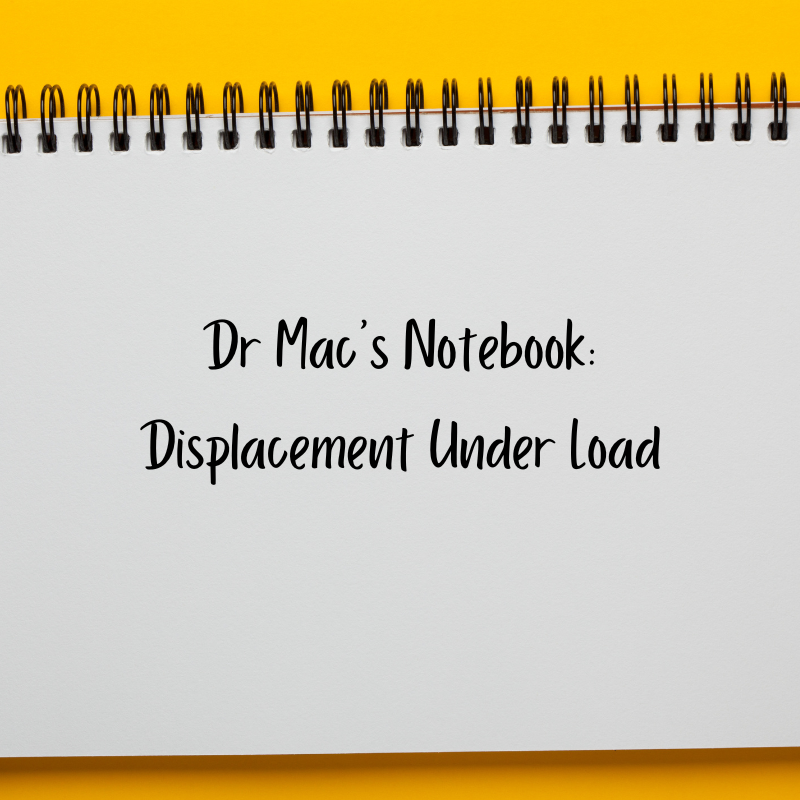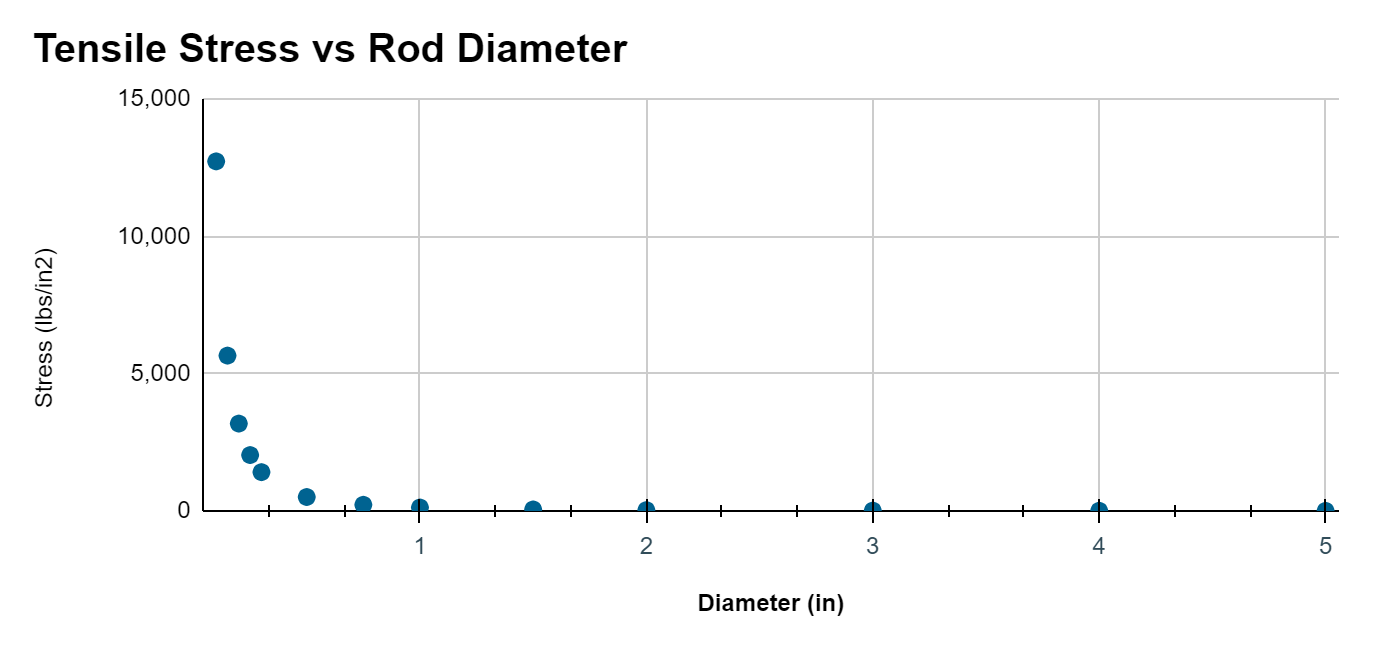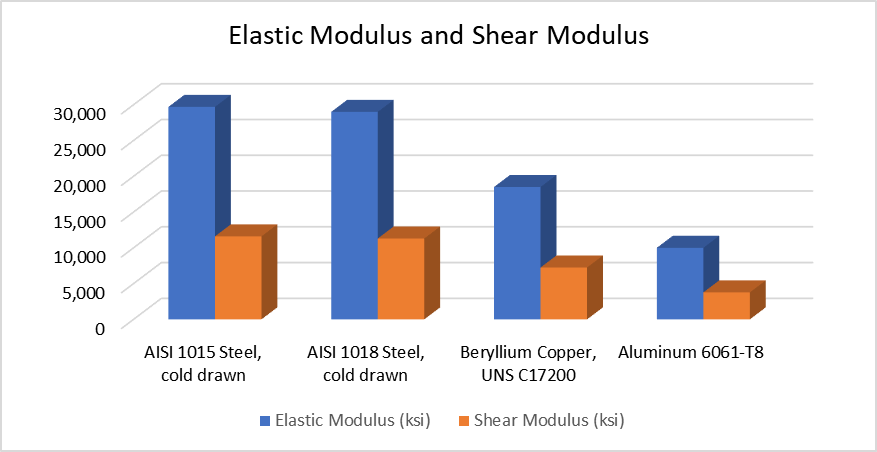# Shop Talk Blog

The Official Blog for Texas Final Drive

In a previous post, we talked about basic mechanical stress -- but this one is going a step further and looking at the relationship between stress, deformation, and strain -- all of which affect how metal parts break.Here are some other Shop Talk Blog posts you might be interested in:

## Springs and Displacement

Let’s start by talking about springs. We know that the harder we pull on a spring, the more it will stretch. And there is a really simple formula to represent that. Let’s say

• x is how much we stretch the spring
• k is the spring rate, which represents how elastic the spring is
• F is the force we apply

F = k ･x

Suppose we have a high carbon spring with an overall length of 2” and a spring rate k = 91.08 lb/in. The k value tells us that we’d need to apply a little over 90 lbs to stretch the spring by one inch. So let’s say we’ve stretched that spring by 1.5 inches. How much force did we use?

F =k ･x = 91.08 ･1.5 = 136.6 lbs

Now let’s look at it a different way. Suppose we apply 200 lbs in tension. How far would the spring stretch?

F = k ･x

200 = 91.08 ･x

Using a little algebra, we can change the equation to find x (we do this by dividing both sides by 91.08):

x = 200 / 91.08

x = 2.20 inches

If we apply 200 lbs, the spring stretches by 2.20 inches. How much the spring stretches is referred to as its displacement -- it’s change in length. The spring's new length is the displacement added to its original length. In the case of this spring, that will be a total of 2” + 2.20” = 4.20”.

## Metal and Displacement

We discussed this in the previous post: how much a metal changes shape under a load is related to its elastic modulus, which is similar to a spring constant. Let’s look at an equation for how a metal rod would stretch under a tensile load. To do this, we need to review a few equations and definitions.

stress = force / area

Stress is how concentrated a force F is as it is applied over an area. So the smaller the area, the higher the stress for the same force. Let’s look at the chart below.For small-diameter rods, the stress is significantly higher than for larger-diameter rods. And that makes sense: if we apply a high tensile force to a small rod and a large rod, both made of the same material, we expect to see more deformation with the larger rod --  and we'd expect the smaller diameter rod to fail first.

## Stress and Displacement

When investigating the displacement (change in length) for metals and plastics, we need to use the stress (concentration of force) and the elastic modulus. If we use E for the elastic modulus for finding the displacement of a rod undergoing tensile stress, here’s that formula:

displacement = (stress length) / E

You can think of E (the Elastic Modulus) as the inverse of the spring constant. So the larger the value of E, the more stress -- and force -- it takes to change its length. Now let's take a look at E.

## Elastic Modulus

Here is a chart that shows how the elastic modulus (and shear modulus) vary for different metals.But wait … steel has a higher elastic modulus than aluminum? That's true! It takes MORE FORCE to stretch a steel rod than an aluminum rod of the same size.

## Conclusion

We know that metals stretch at different rates, and how much they stretch relates to their modulus of elasticity. So what does this have to do with your final drive motor or pump? First, the engineers chose the materials used in their manufacture for good reasons. But this particular serves as a building block for our next topic: what loads metals fail at. So stay tuned!Topics: Final Drive Failure